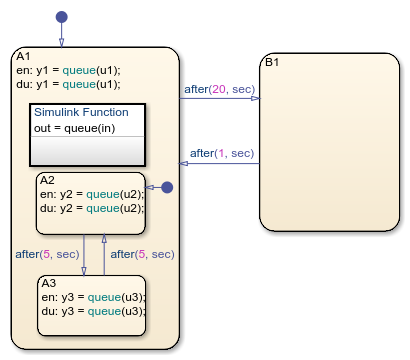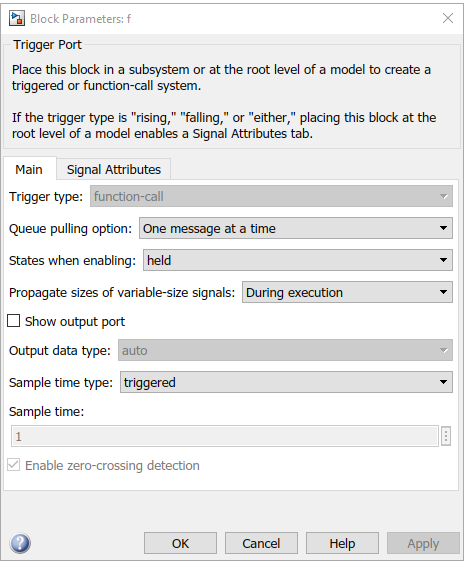## Bind a Simulink Function to a State

Simulink® functions are not supported in standalone Stateflow® charts in MATLAB®.

When a Simulink function resides inside a state, the function binds to that state. Binding results in the following behavior:

• Function calls can occur only in state actions and on transitions within the state and its substates.

• When the state is entered, the function is enabled.

• When the state is exited, the function is disabled.

For example, the following Stateflow chart shows a Simulink function that binds to a state.Since the function `queue` resides in state `A1`, the function binds to state `A1`.

• State `A1` and its substates `A2` and `A3` can call the function `queue`, but state `B1` cannot.

• When state `A1` is entered, `queue` is enabled.

• When state `A1` is exited, `queue` is disabled.

### Control Subsystem Variables When the Simulink Function Is Disabled

If a Simulink function binds to a state, you can hold the subsystem variables at their values from the previous execution or reset the variables to their initial values. To choose the desired behavior for your subsystem, follow these steps:

1. In the Simulink function, double-click the trigger port to open the Block Parameters dialog box.

2. Select an option for States when enabling.

OptionDescription
`held`Holds the values of the subsystem variables from the previous execution
`reset`Resets the subsystem variables to their initial values

### Binding a Simulink Function to a State

This example shows how a Simulink function behaves when bound to a state.The function `queue` contains a block diagram that increments a counter by 1 each time the function executes.The Block Parameters dialog box for the trigger port appears as follows.In the dialog box, setting Sample time type to `periodic` enables the Sample time field, which defaults to 1. These settings tell the function to execute for each time step specified in the Sample time field while the function is enabled.

If you use a fixed-step solver, the value in the Sample time field must be an integer multiple of the fixed-step size. This restriction does not apply to variable-step solvers. For more information, see Compare Solvers (Simulink).

#### Simulation Behavior of the ChartWhen you simulate the chart, the following actions occur.

1. The chart takes the default transition to state `A1`, and the local data `u1` is set to 1.

2. When `A1` is entered, the function `queue` is enabled.

3. Function calls to `queue` occur until the condition `after(5, sec)` is true.

4. Once the condition is true, the transition from state `A1` to `B1` occurs.

5. When `A1` is exited, the function `queue` is disabled.

6. After two more seconds pass, the transition from `B1` to `A1` occurs.

7. Steps 2 through 6 repeat until the simulation ends.

#### Function Behavior When Variables Are Held

If you set States when enabling to `held`, the output `y1` is as follows.When state A1 becomes inactive at t = 5, the Simulink function holds the counter value. When A1 is active again at t = 7, the counter has the same value as it did at t = 5. Therefore, the output `y1` continues to increment over time.

#### Function Behavior When Variables Are Reset

If you set States when enabling to `reset`, the output `y1` is as follows.When state A1 becomes inactive at t = 5, the Simulink function does not hold the counter value. When A1 is active again at t = 7, the counter resets to zero. Therefore, the output `y1` resets too.

## SupportGet trial now